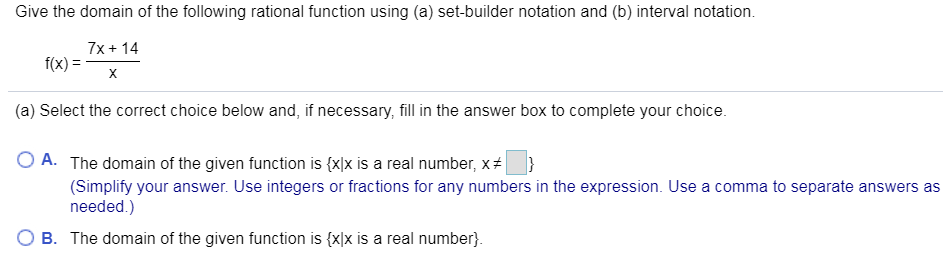# Give the domain of the following rational function using (a) set-builder notation and (b) interval notation 7x14 f(x) X (a) Select the correct choice below and, if necessary, fill in the answer box to complete your choice. O A. The domain of the given function is {x]x is a real number, x } (Simplify your answer. Use integers or fractions for any numbers in the expression. Use a comma to separate answers as needed.) B. The domain of the given function is {xlx is a real number

Questionhelp_outlineImage TranscriptioncloseGive the domain of the following rational function using (a) set-builder notation and (b) interval notation 7x14 f(x) X (a) Select the correct choice below and, if necessary, fill in the answer box to complete your choice. O A. The domain of the given function is {x]x is a real number, x } (Simplify your answer. Use integers or fractions for any numbers in the expression. Use a comma to separate answers as needed.) B. The domain of the given function is {xlx is a real number fullscreen

### Want to see this answer and more?

Experts are waiting 24/7 to provide step-by-step solutions in as fast as 30 minutes!*